#Calculus Worksheets

## Differential Equations Worksheets

Here is a graphic preview for all of the Differential Equations for Calculus Worksheets. You can select different variables to customize these Differential Equations for Calculus Worksheets for your needs. The Differential Equations for Calculus Worksheets are randomly created and will never repeat so you have an endless supply of quality Differential Equations for Calculus Worksheets to use in the classroom or at home. We have slope field, separable equation, differential equation, and exponential growth worksheets.

Our Differential Equations for Calculus Worksheets are free to download, easy to use, and very flexible.

These Differential Equations for Calculus Worksheets are a good resource for students in high school.

Click here for a Detailed Description of all the Differential Equations for Calculus Worksheets.

## Quick Link for All Differential Equations for Calculus Worksheets

Click the image to be taken to that Differential Equations for Calculus Worksheets.

##### Solving Separable Equations Worksheets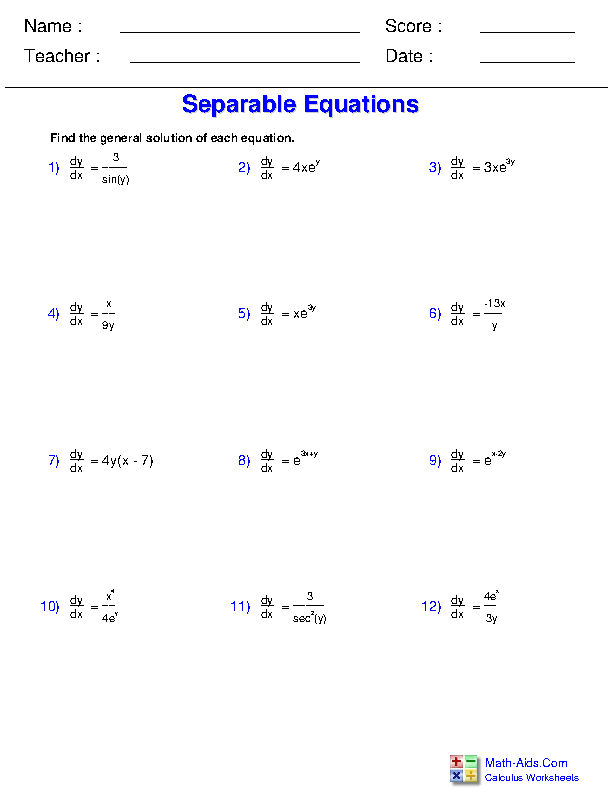##### Drawing Slope Fields Worksheets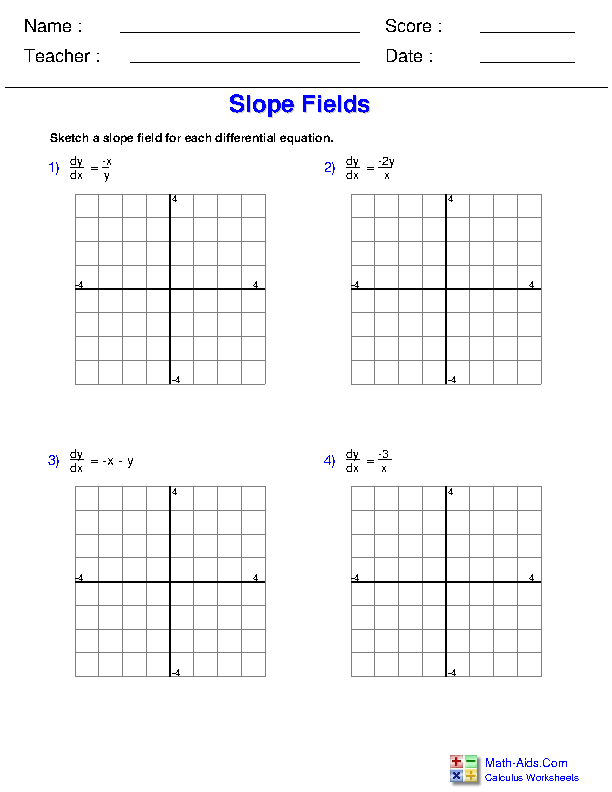##### Differential Equation Worksheets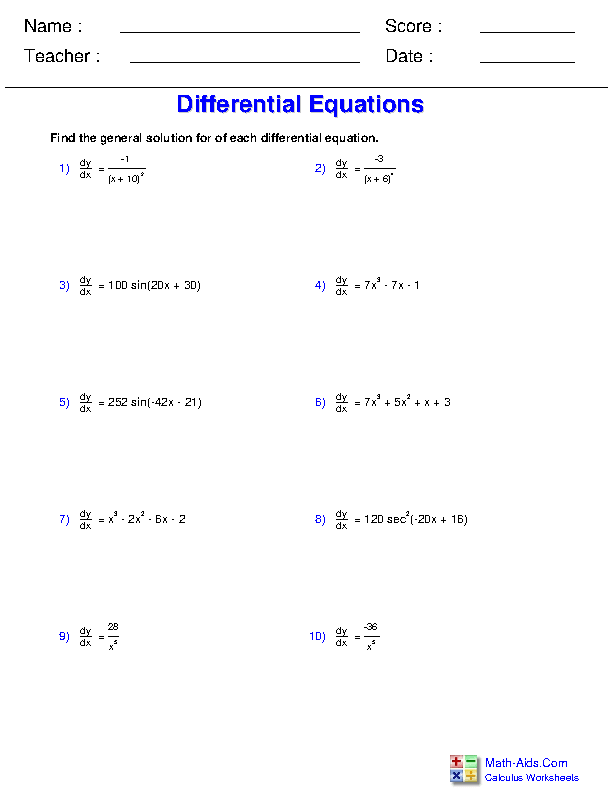##### Differential Equation Slope Field Worksheets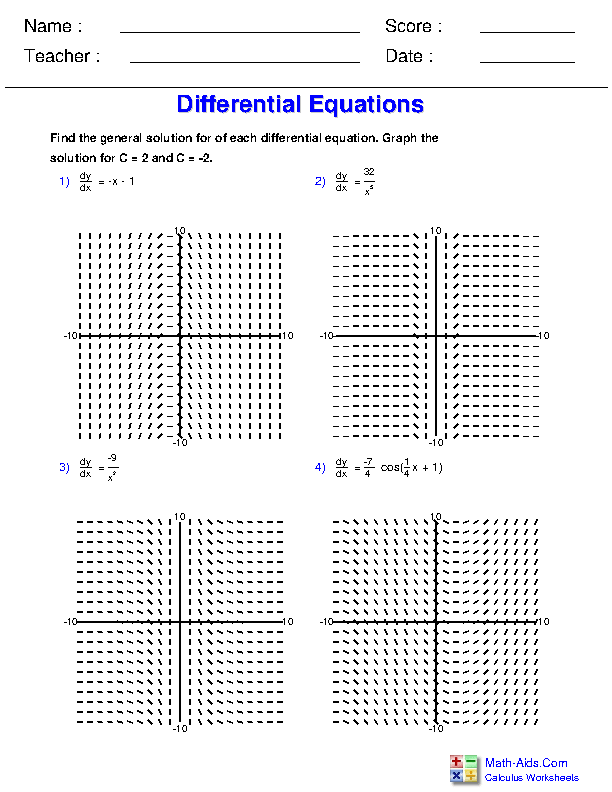##### Exponential Growth and Decay Worksheets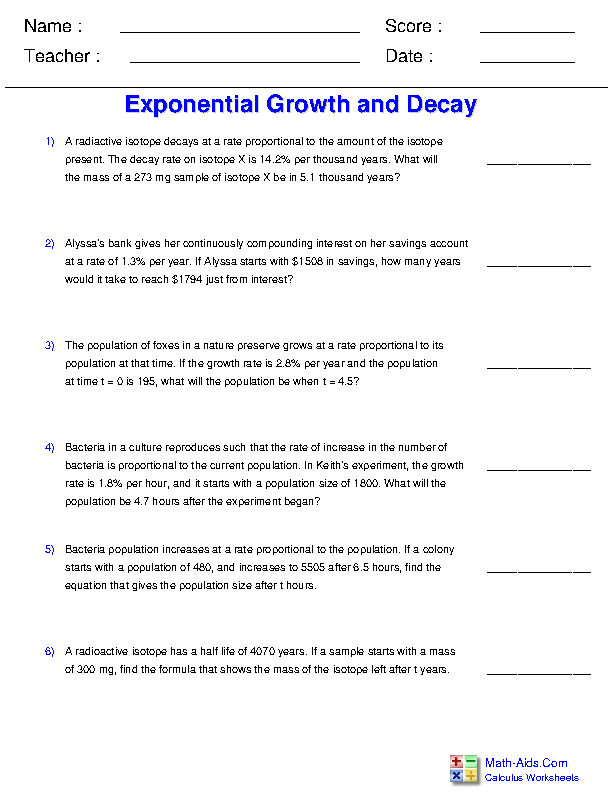Recommended Videos

## Detailed Description for All Differential Equations for Calculus Worksheets

Solving Separable Equations Worksheets
This Calculus - Differential Equations Worksheet will produce problems that involve solving separable differential equations.

Drawing Slope Fields Worksheets
This Calculus - Differential Equations Worksheet will produce problems that involve drawing slope fields of differential equations.

Differential Equation Worksheets
This Calculus - Differential Equations Worksheet will produce problems that involve approximating the area under a curve by drawing and calculating Riemann sums.

Differential Equation Slope Field Worksheets
This Calculus - Differential Equations Worksheet will produce problems that deal with using a slope field to graph a differential equation.

Exponential Growth and Decay Worksheets
This Calculus - Differential Equations Worksheet will produce word problems that deal with finding equations for exponential growth or decay.